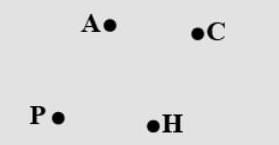Courses
Courses for Kids
Free study material
Free LIVE classes
MoreLIVE
Join Vedantu’s FREE Mastercalss

# With a sharp tip of the pencil, mark four points on a paper and name them by the letters A, C, P, H. Try to name these points in different ways. One such way could be this.Verified
359.1k+ views
Hint: Approach by fixing each point to all locations such that one letter is considered at each true. We have to find all the possible naming methods applicable without repetition.

First, consider the letter A. For A, we have four different positions available amongst which we can select one.
So, number of ways of arranging A, $\therefore A=4$
Since, we have placed A so we are left with three other letters, that is C, P, H.

Now, consider the letter C. Since, one place is occupied by A, so we have three different positions available amongst which we can select one.
So, number of ways of arranging C, $\therefore C=3$
Since we have placed A & C. So, we are left with two other letters, that is P, H.

Again, consider the letter H. It has only two places for solution amongst four places.
So, number of ways of arranging H, $\therefore H=2$
We have fixed three letters up till now and left with only P.

At last consider P but we have only one left space for placing P.
So, no of ways of arranging P, $\therefore P=1$

As we know that for two events ${{a}_{1}}\text{ and }{{a}_{2}}$ the total outcomes are${{a}_{1}}\times {{a}_{2}}$ by multiplication principle.

By using the rule of multiplication principle:
\begin{align} & A\times C\times H\times P=4\times 3\times 2\times 1 \\ & =24 \\ \end{align}

Therefore, the total outcomes are 24.

Note: Common confusion about application of rule of addition and rule of multiplication. Here, we cannot use the rule of addition because events are not disjoint.
One simpler method of solving this question is by using permutation.
Total number of outcomes $={}^{n}{{P}_{r}}$
Here, n=4 and r=4.
\begin{align} & \therefore {}^{4}{{P}_{4}}=\dfrac{4!}{\left( 4-4 \right)!} \\ & \Rightarrow \dfrac{4!}{0!}=24 \\ \end{align}
Last updated date: 21st Sep 2023
Total views: 359.1k
Views today: 8.59k